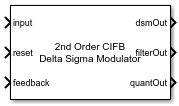# Delta Sigma Modulator

Model a discrete delta sigma modulator based ADC

Since R2021b

•Libraries:
Mixed-Signal Blockset / ADC / Building Blocks

## Description

Use the Delta Sigma Modulator block to model a discrete delta sigma modulator based ADC using a set of different architectures. The block supports these architectures:

You can model an ADC of orders from two to six. You can also model circuit based noise.

## Ports

### Input

expand all

Data Types: `double`

Reset signal for Delta Sigma Modulator block, specified as a scalar.

Data Types: `double`

### Output

expand all

Digital output signal, returned as a scalar.

Data Types: `fixed point` | `single` | `double` | `int8` | `int16` | `int32` | `uint8` | `uint16` | `uint32` | `Boolean`

## Parameters

expand all

Architecture of the delta sigma modulator, specified as:

• `CIFB` — cascade of feedback integrators.

• `CRFB` — cascade of feedback resonators.

• `CIFF` — cascade of feed-forward integrators.

• `CRFF` — cascade of feed-forward resonators.

#### Programmatic Use

 Block parameter: `dsmArchitecture` Type: character vector Values: `CIFB` | `CRFB` | `CIFF` | `CRFF` Default: `CIFB`

Order of delta sigma modulator. You can choose between orders two to six.

#### Programmatic Use

 Block parameter: `dsmOrder` Type: character vector Values: `2nd order` | `3rd order` | `4th order` | `5th order` | `6th order` Default: `2nd order`
Input Parameters

Sampling frequency of the delta sigma modulator, specified as a positive real scalar in Hz.

#### Programmatic Use

 Block parameter: `SamplingFrequency` Type: character vector Values: positive real scalar Default: `128e3`

Number of quantizer levels in delta sigma modulator, specified as a positive real scalar.

#### Programmatic Use

 Block parameter: `NumberLevels` Type: character vector Values: positive real scalar Default: `2`

Range of the quantizer output in the delta sigma modulator architecture, specified as a vector with real elements.

#### Programmatic Use

 Block parameter: `QuantizerOutput` Type: character vector Values: real valued vector Default: `[-1;1]`

Feedback coefficients from the quantizer or feed-forward coefficients to the quantizer, specified as a vector with real elements.

#### Programmatic Use

 Block parameter: `a` Type: character vector Values: real valued vector Default: `[0.1601 0.6515]`

Resonator coefficients, specified as a vector with real elements.

#### Programmatic Use

 Block parameter: `g` Type: character vector Values: real valued vector Default: `[0.0]`

Feed-in coefficients from the modulator input to each integrator, specified as a vector with real elements.

#### Programmatic Use

 Block parameter: `b` Type: character vector Values: real valued vector Default: `[0.1601 0.6515 1]`

Integrator inter-stage coefficients, specified as a vector with real elements.

#### Programmatic Use

 Block parameter: `c` Type: character vector Values: real valued vector Default: `[1 1]`

Click to populate the default values of the coefficients 'a', 'g', 'b', and 'c'. The block calculates the coefficient values based on the architecture and order of the delta sigma modulator.

Noise

Enable noise calculations in the delta sigma modulator ADC.

Ratio of signal power to noise power, specified as a nonnegative real scalar in dB.

#### Programmatic Use

 Block parameter: `SNR` Type: character vector Values: nonnegative real scalar Default: `75`

Power of the signal at the input of the delta sigma modulator, specified as a real scalar in watts.

#### Programmatic Use

 Block parameter: `InputPower` Type: character vector Values: real scalar Default: `0.125`

Bandwidth of the delta sigma modulator system,

#### Programmatic Use

 Block parameter: `BandWidth` Type: character vector Values: nonnegative real scalar Default: `1000`

## Version History

Introduced in R2021b